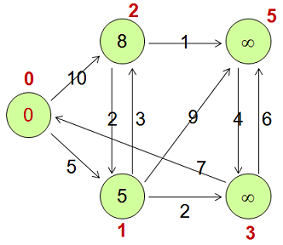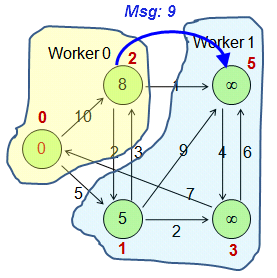MaxCompute Graph is a processing framework designed for iterative graph computing. Graph computing jobs use graphs to build models. A graph is a collection of vertices and edges that have values.

MaxCompute Graph allows you to perform the following operations on graphs:
• Change the value of a vertex or edge.
• Add or remove a vertex.
• Add or remove an edge.
Note When you edit a vertex or edge, you must use code to maintain the relationships between vertices and edges.

MaxCompute Graph performs iterations to edit graphs, enable graphs to evolve, and obtain analysis results. Typical applications include the PageRank, single source shortest path (SSSP) algorithm, and k-means algorithm. You can use SDK for Java provided by MaxCompute Graph to compile graph computing programs.

Terms

• Graph: an abstract data structure used to represent the relationships between objects. Vertices and edges are used in graphs. Vertices represent objects, and edges represent the relationships between the objects. The data described in graphs is called graph data.
• Vertex: represents an object in a graph.
• Edge: a single directed edge that represents the relationship between objects in a graph. An edge consists of a source vertex ID, a destination vertex ID, and the data associated with the edge.
• Directed graph: a graph in which edges have directions. Two vertices on an edge represent different objects. For example, Page A is connected to Page B. In directed graphs, edges are classified into outgoing edges and incoming edges.
• Undirected graph: a graph in which edges do not have directions, such as common users in a user group.
• Outgoing edge: a directed edge on which the current vertex is the origin.
• Incoming edge: a directed edge on which the current vertex is the destination.
• Degree: the number of edges that connect to a vertex.
• Outdegree: the number of outgoing edges that connect to a vertex.
• Indegree: the number of incoming edges that connect to a vertex.
• Superstep: an iteration of a graph.

Graph data structure

MaxCompute Graph processes directed graphs. MaxCompute provides only a two‑dimensional table storage structure. Therefore, you must resolve graph data into two‑dimensional tables and store them in MaxCompute. You can resolve the graph data based on your business requirements.

During graph computing and analytics, use custom GraphLoader to convert two-dimensional table data into vertices and edges that are applicable to MaxCompute Graph.
• The structure of a vertex is <ID, Value, Halted, Edges>. Parameters:
• ID: the ID of the vertex.
• Value: the value of the vertex.
• Halted: specifies whether the iteration of the vertex is terminated.
• Edges: the edges that start from the vertex.
• The structure of an edge is <DestVertexID, Value>. Parameters:
• DestVertexID: the ID of the destination vertex.
• Value: the value of the edge.For example, the preceding figure can be expressed in the following two‑dimensional table.
Vertex <ID, Value, Halted, Edges>
v0 <0, 0, false, [<1, 5 >, <2, 10 >]>
v1 <1, 5, false, [<2, 3>, <3, 2>, <5, 9>]>
v2 <2, 8, false, [<1, 2>, <5, 1 >]>
v3 <3, Long.MAX_VALUE, false, [<0, 7>, <5, 6>]>
v5 <5, Long.MAX_VALUE, false, [<3, 4 >]>

Logic of a Graph program

A Graph program performs the following operations: graph loading, iterative computing, and iteration termination.

1. Graph loading: MaxCompute Graph calls the custom GraphLoader to resolve the records in the input table into vertices or edges.
2. Partitioning: MaxCompute Graph calls the custom Partitioner to partition the vertices and distributes the partitions to the related workers. By default, MaxCompute Graph partitions the vertices based on the hash values of the vertex IDs modulo the number of workers.In the preceding figure, the number of workers is 2. Vertex 0 and Vertex 2 are distributed to Worker 0 because the result of vertex IDs modulo 2 is 0. Vertex 1, Vertex 3, and Vertex 5 are distributed to Worker 1 because the result of vertex IDs modulo 2 is 1.
2. Iterative computing

An iteration is called a superstep. During an iteration, the program traverses all non-halted vertices or all vertices that receive messages, and calls the compute(ComputeContext context, Iterable messages) method. For non-halted vertices, the value of the Halted parameter is false. Halted vertices are automatically activated after they receive messages.

The compute(ComputeContext context, Iterable messages) method can be used to perform the following operations:
• Process the messages that are sent by the previous superstep to the current vertex.
• Edit a graph as required:
• Change the values of vertices or edges.
• Send messages to some vertices.
• Add or remove vertices or edges.
• Use an aggregator to collect information and update global information. For more information, see Aggregator.
• Set the current vertex to the halted or non-halted state.
• Enable MaxCompute Graph to asynchronously send messages to the related workers in each superstep. The messages are then processed in the next superstep.
3. Iteration termination
An iteration is terminated if one or more of the following conditions are met:
• All vertices are in the halted state (the value of the Halted parameter is true), and no new messages are generated.
• The maximum number of iterations is reached.
• The terminate method of an aggregator returns true.
Pseudocode of the Graph program:
for each record in input_table {
}
// 2. setup
WorkerComputer.setup();
for each aggr in aggregators {
aggr.createStartupValue();
}
for each v in vertices {
v.setup();
}
// 3. superstep
for (step = 0; step < max; step ++) {
for each aggr in aggregators {
aggr.createInitialValue();
}
for each v in vertices {
v.compute();
}
}
// 4. cleanup
for each v in vertices {
v.cleanup();
}
WorkerComputer.cleanup();